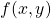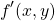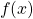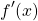# Category Archives: Mechanics

Study has been so busy this year, I've failed in keeping an up-to-date account of how it's been going. Here's a very quick run-though:

Assignment 2:

• First and second-order differential equations.
• Vector algebra and statics. (There are two blocks connected with taut string on a ramp and nothing is moving, what are the forces?)
• Dynamics. (A block is sliding down a ramp with a certain friction, what are the forces?)

Assignment 3:

• Matrices and determinants.
• Eigenvalues and eigenvectors.
• Systems of linear differential equations. (solving simultaneous differential equations).
• Functions of several variables. (andand).

Assignment 4:

• Mathematical modelling. (Introduction to writing a mathematical modelling paper).
• Oscillations and energy. (Forces in a spring system, and potential energy).
• Forcing, damping an resonance. (Forces in a system of springs and dampers).
• Normal modes. (Oscillations of particles in a spring system).

Assignment 5:

• Systems of differential equations. (Equilibrium points of two differential equations and use of the Jacobian matrix).
• Fourier series.
• Partial differential equations.

Assignment 6:

• Vector calculus. (Scalar fields and gradients).
• Further vector calculus. (Conservative vector fields, curl, and divergence).
• Multiple integrals. (Area and volume integrals).

Assignment 7:

• Writing a 3000-word mathematical modelling paper.

All the above are now complete, and I'm currently working through the last few units. Namely:

• Systems of particles.
• Circular motion.
• Rotating bodies and angular momentum.

There's a requirement for me to submit one assignment per month, from October to May. These double-credit modules are hectic...

Out of all of the above, Assignment 7 had to be the most nerve-racking. Most assignments normally read like exam papers, though for Assignment 7 I had to write a report. So not only had I never written a report like this on this course before, I'd never written a report like this ever! Results are due in a couple of weeks, so based on the outcome I'll be keen to break down where I went wrong.

Favourite bits from the above have to include my first ever hand-calculation of a Fourier series in Assignment 5, and a question where I had to prove a result of Archimedes (287-212 BCE) regarding relative volumes, using modern calculus in Assignment 6. I'd also always wanted to know more about the construction of a Jacobian, so Assignment 5 was good for applying my new knowledge.

# Unit 1 COMPLETE!

My first unit is done! I managed to find time to complete the first assignment too. This is timely, as the course officially starts tomorrow.

This unit was interesting. Looking at the title "differential equations" I thought "Oh, okay, fine. I've done this before". Then opening up the book, I realised "Oh wait. I haven't quite done THIS before". That's when the fear crept in... how much of a learning curve would I be facing in this new unit?!

Forging through, I had to concede defeat on one or two examples, but I came out the other end confident about all the material I'd covered. Only now I've completed the assignment do I feed confident about my ability on the subject matter, but it's also one of those areas where I need to keep up the practise.

All too often, it's the first unit that you return to at revision time and think "what IS all this?!". It's been hinted at that themes of second order differential equations reoccur throughout the module so I'm hoping I'll stay in practise.

So I'll give the assignment a final proof-read and then submit it tomorrow on the first official day of the module. Good start.

# Book Arrival

My books finally arrived.

I've got nine months to get all of that inside my brain. -but then I will also need to be able to write answers to questions on the subject matter at close to the speed of sound by the time of the exam...

# Mechanics

After taking a much needed break from study for a year, today is the day I start my next module! Mathematics methods, models and modelling (which essentially amounts to "Mechanics").

Over the past year, I've been trying to keep my brain moderately agile by reading up on automata theory, which in some respects is a continuation of group theory from my previous Pure Mathematics module. If I ever have time, I'll write some findings up here about it. Though given I haven't even found time to write up my results of my Analysis work from my last module, this may too fall by the wayside!

Though despite the fact that my brain hasn't been entirely switched off over the past year, I'm still a bit nervous about starting this new module given that I haven't looked at calculus in such a long time. Here's hoping it all comes flooding back!

It seems that in this module there's yet another type of mathematics software that's been introduced. This one is called Maxima. It appears to perform the same role as Mathematica or Maple. Though it's another type of software, it shouldn't take too long to become familiar with it.

First section is on first and second-order differential equations, and the first assignment appears to contain a merciful count of three questions. Time to dive in!